# Illustrator Tutorial: Vector CompassHave you ever thought of how to draw a vector compass in Illustrator? This tutorial with cover all the steps to draw a nice vector compass with a magnifying glass. Hope you have fun learning this tutorial.

1. First, let us open a new document. To open a new document you will go to File > New. When you click on New a new document will open. As shown in the image above, we will name the file and then we will input the size of the artboard. One thing that we should always remember is to set the color mode in the document to RGB, so that the image looks like an original image.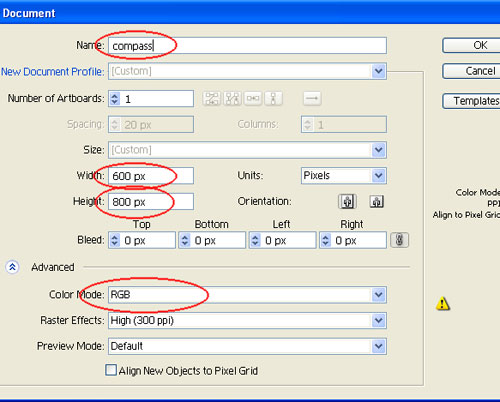2. First, make a circle.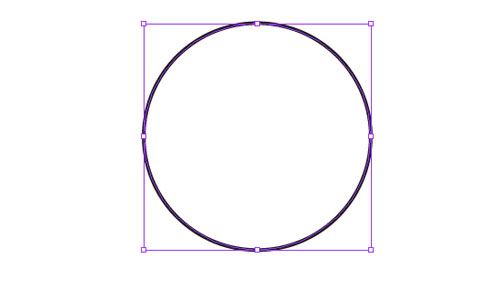3. Make one more circle inside the first circle.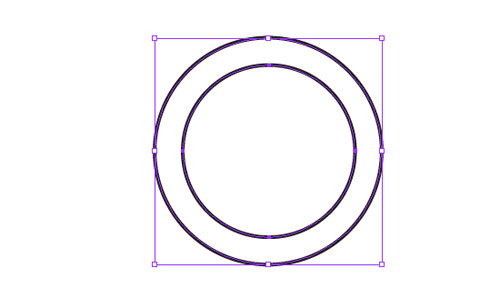4. After making the circles, select the two circles and make a compound. Refer to the image shown below. Go to Object > Compound Path > Make.5. After making the compound, the circle is cropped from the middle. Lets go to next step and fill this shape with a radial gradient color. The value of the gradient is given below.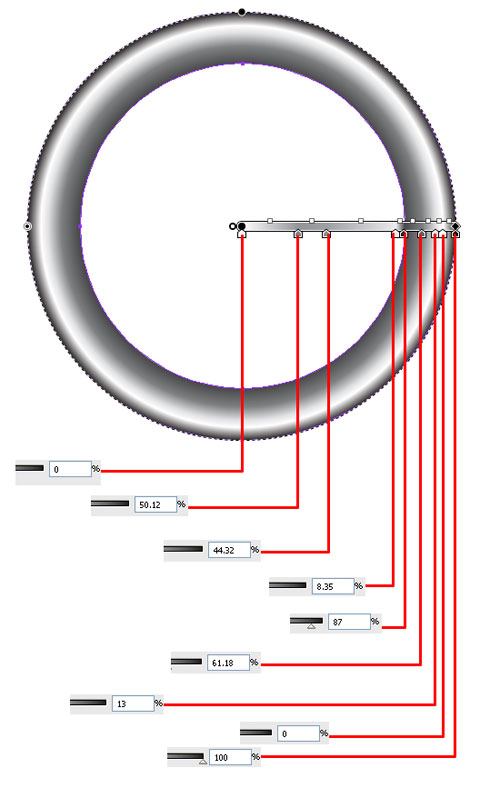6. After filling the gradient, our image will look something like the image shown above.

7. Let us then proceed further and make a shape, as shown below and marked by a red arrow.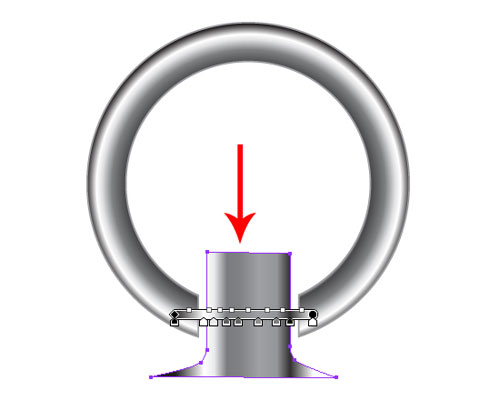8. Fill this image with gradient color. The value of the gradient is given in the image below.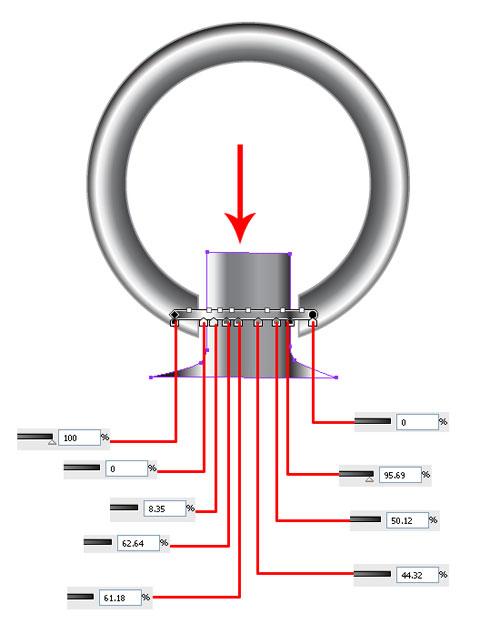9. Let us make the shape shown in the image below and fill this image with the same gradient color used above. Rotate the gradient by 180 degrees. By doing so, the gradient will be reversed.10. Place the lines filled with gradient on this shape marked by the red arrow. You can fill the lines with the gradient of your choice.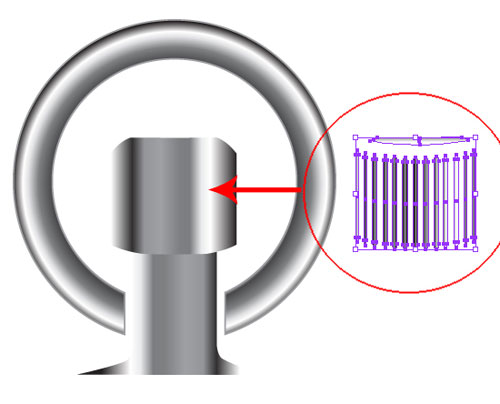11. After placing the shape, our image will look like this.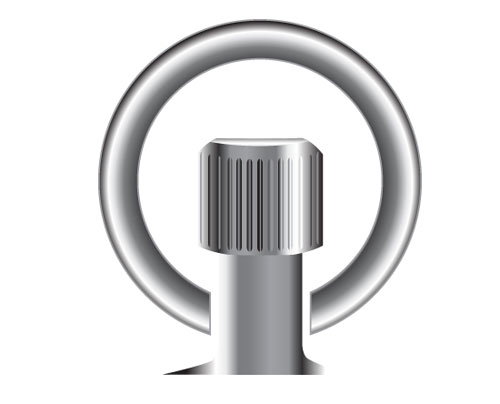12. Let us now proceed towards the bottom part of the compass. Make a circle shape as shown in the image below.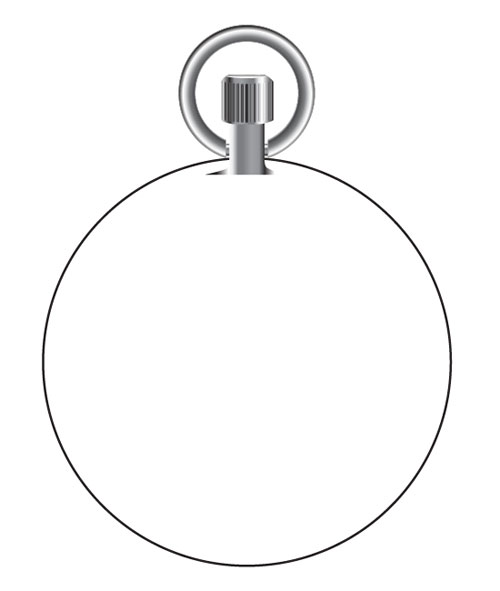13. Fill this shape with the gradient color with values shown in the image below.14. Next, let’s make one more circle inside this circle. Fill it with the gradient shown in the image above, but change the orientation of the grading. As you can see, the gradient’s line is straight at the moment. Now, selecting the gradient tool, we’ll drag this line again so the orientation of the gradient changes.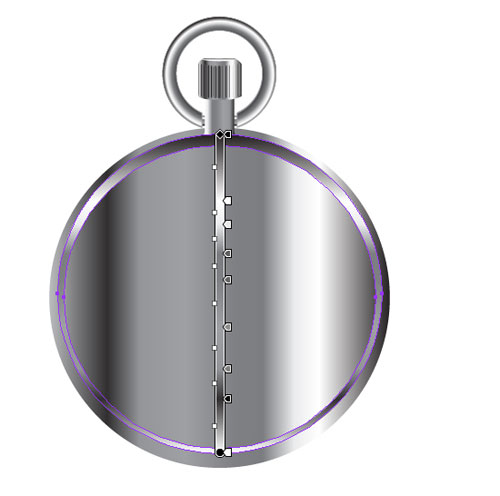15. To Proceed to the next step, make one more circle inside the shape of the circle and fill it with random gradient color. You can choose any gradient color for this.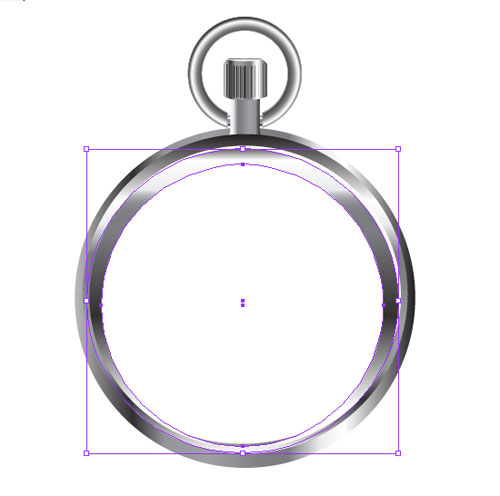16. Now, we have made one part of the compass in silver color. Let us then move on to the next step and make 2 more circles inside this shape. Fill the circles with colors, as shown below.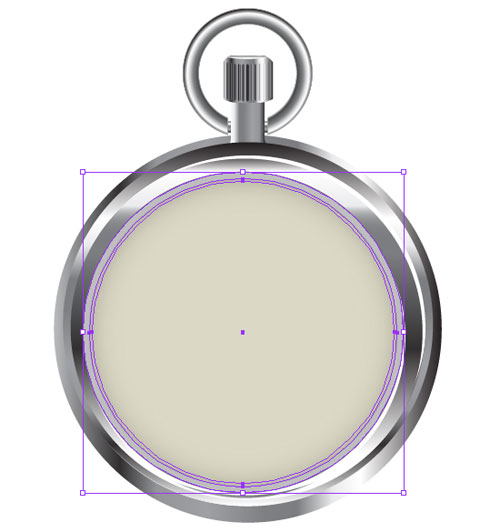17. We will place a circle of black color in the smallest circle. Refer to the image below.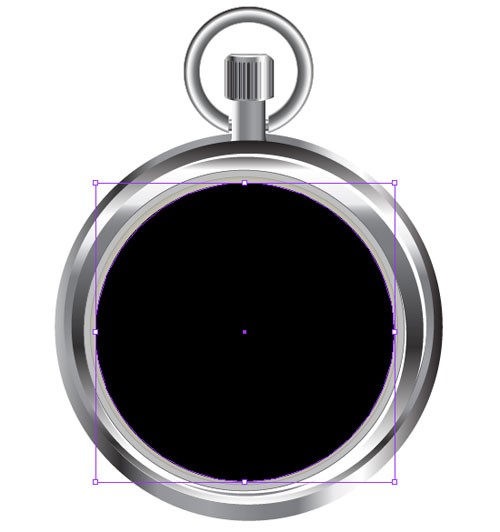18. Now, the basic structure of our compass is complete. Therefore, we are ready to proceed to making the inner part of this compass. You would have seen how numbers are placed in a clock, so we will apply the same method to this part of the compass.19. Now we’ll place outlines in between this black cirlce as shown in the image below.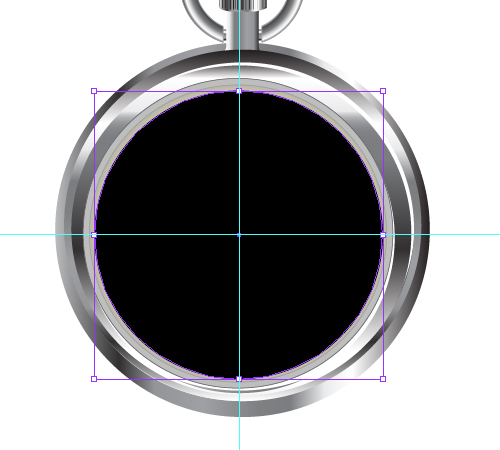20. Now we’ll make a small stroke on the upper side.21. Press R key and drag it’s round point in the centre of the circle.22. As shown in the image.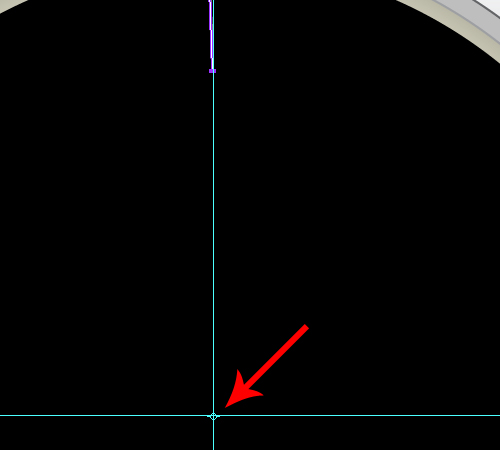23. Now press Alt+Shift and drag this stroke by doing so it will rotate itself.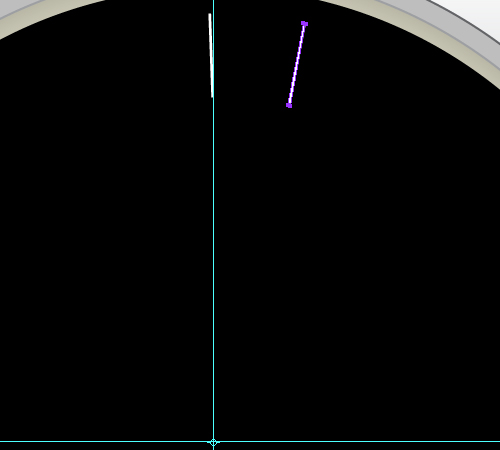24. Press Ctrl+D till the full circle is completed.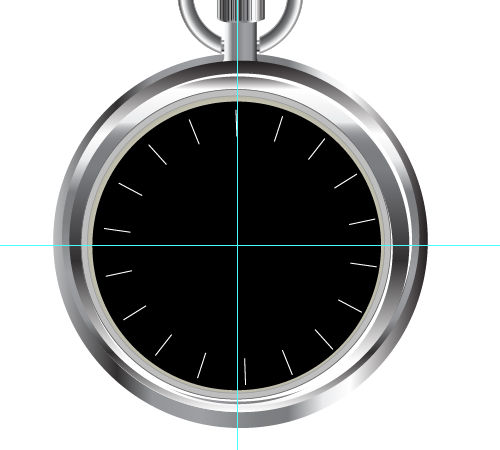25. Similarly we’ll make the other round and use the same technique as mentioned above.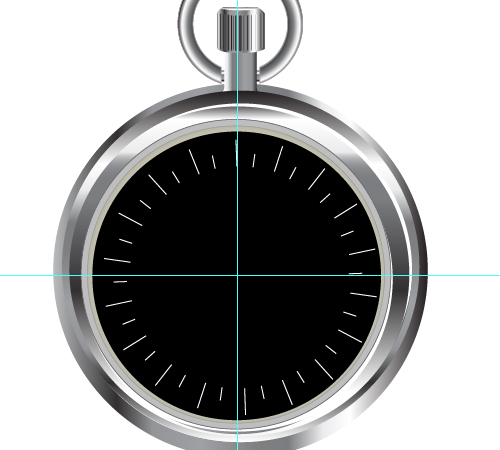26. Lets proceed and insert the 3rd and last lines, we have used the same technique for this too. After this hide the outlines.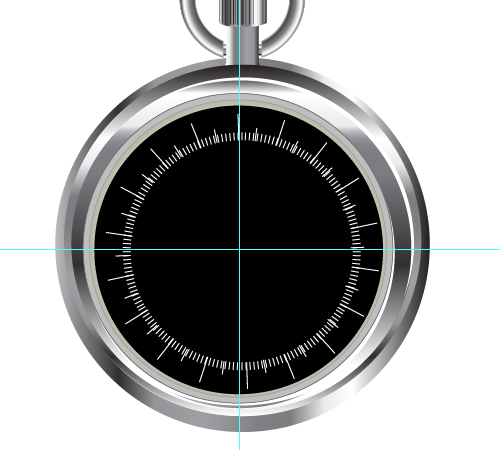27. Once we have finished placing the lines, we will then place the corresponding numbers.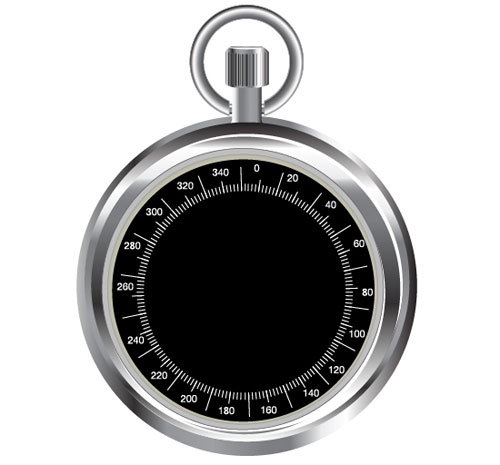28. The next step is making the needles. To make the needles, let’s create a shape, as shown in the image below.29. We’ll fill this shape with gradient with the value given in the image below. Remember that the center part of gradient has 2 nodes; one node is hidden behind the other node, but we have to give the gradient value to both nodes.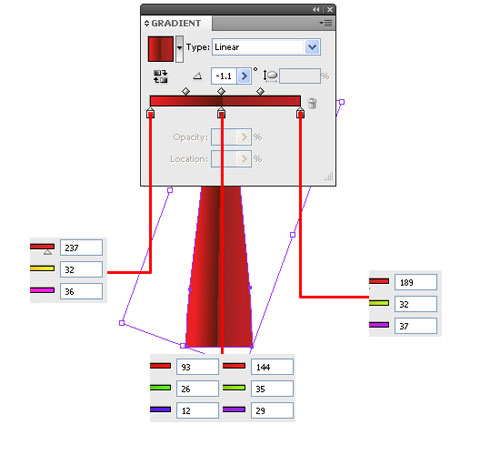30. Now that we have made one side of the needle, we will proceed with other side of the needle. We will only change the color from red to green and it will look like this.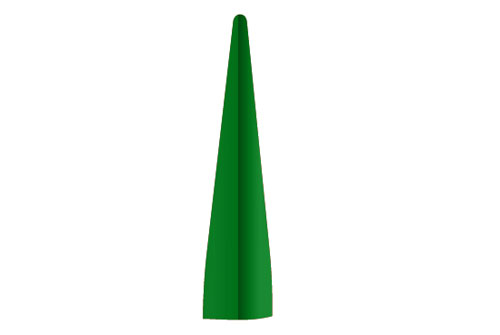31. Let us attach both these needles and place them in the center of the compass. We will also change the rotation of the needles.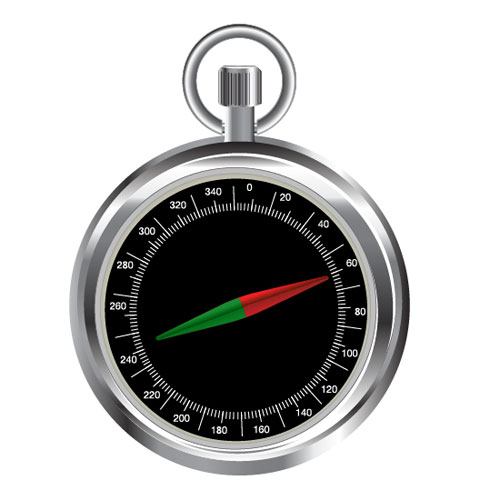32. After placing the needles in the middle of the compass, we will make 2 more circles in the middle of the compass and fill them with the simple gradient. The illustrator will take the default value so we need not give any value for it.33. Let us proceed further and make some needles as shown in the image below.34. Place the shape in the middle of the compass and change the color from black to white. After this, our image will look like this.35. Now, we will place the text at the tip of each white needle, indicating the direction.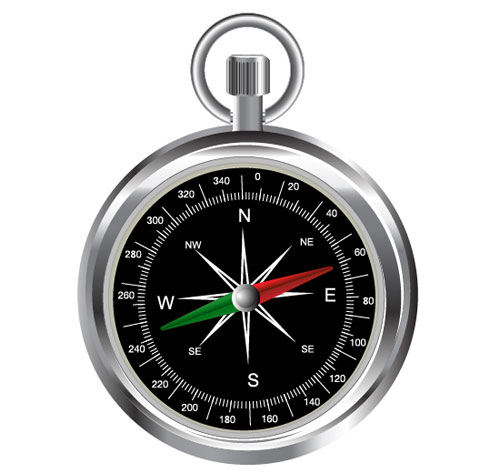36. Now, we will give the compass a glow. To do so, make a shape with the pen tool, as shown in the image below.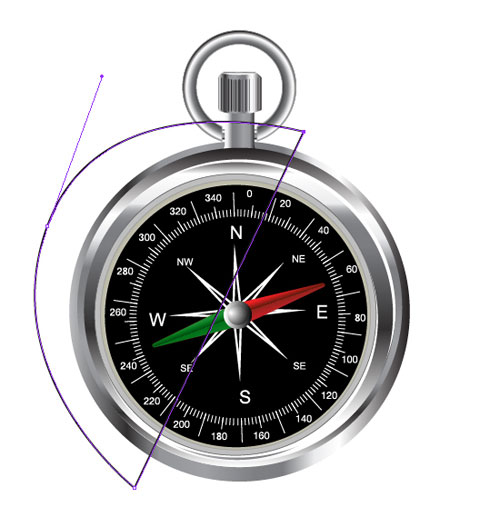37. Select the black circle and press Ctrl + F. By doing so, the black shape will be copied on the shape made by the pen tool. See the image below.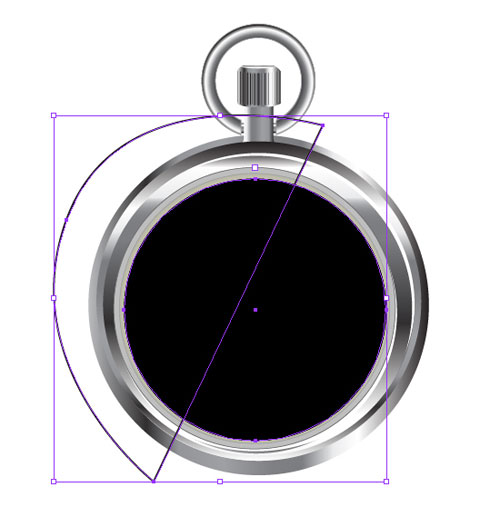38. Go to Window > Pathfinder and you will see a window in front of you. Select the option marked red in the image below.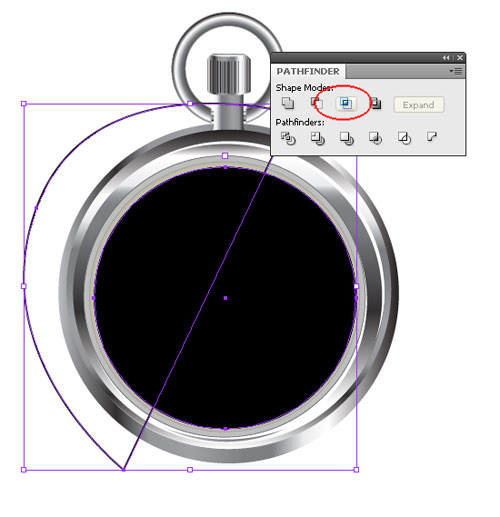39. After selecting the said option, we will see a new shape, in which we will fill with the gradient color. To fill the gradient color, refer to the window shown below. Remember, we have to set the opacity value of black in the gradient to zero.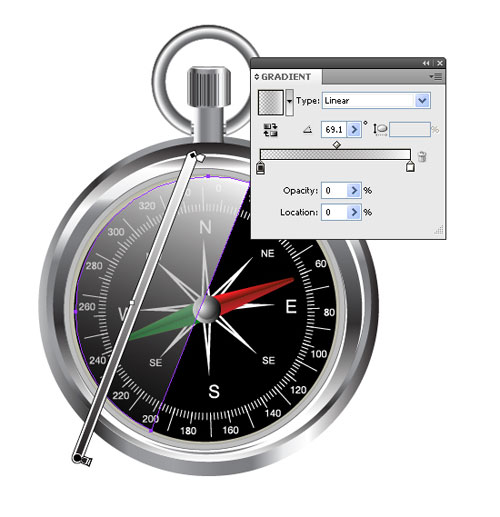40. Notice the image shown below. The compass adjustment clutch is copied and pasted at the lower part.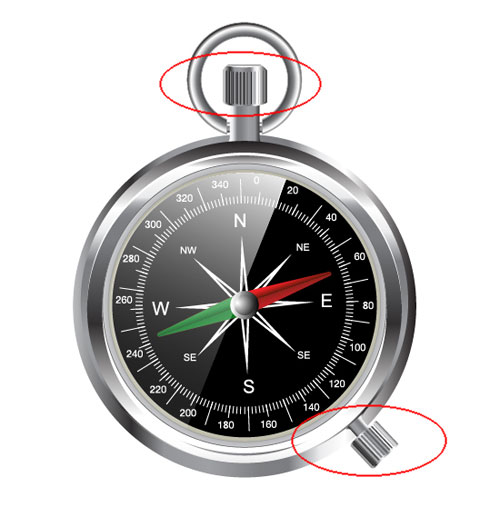### Drawing the Magnifying Glass

41. Now that we have made the compass, let’s start working on the magnifying glass.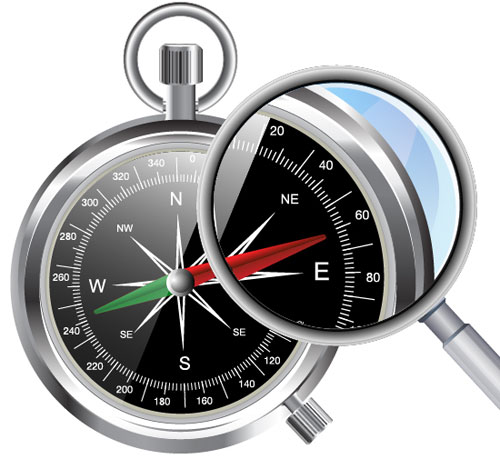42. Let us make a circle and fill it with gradient color. We have to use the same technique for making the circle as we used in making the compass.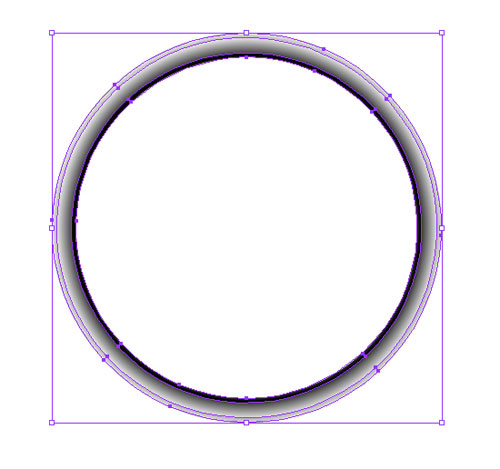43. To make the handle of magnifying glass, make a box and fill it with default gradient color.44. We will also make one more box that will be slightly bigger than the first one. Fill it with gradient color, too.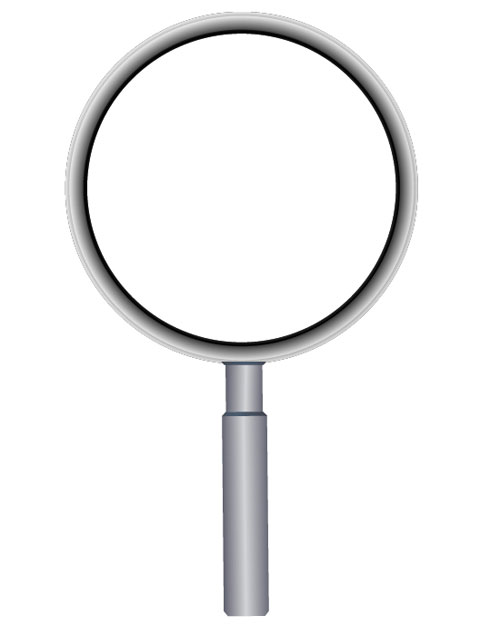45. After making the handle, make 2 more small box shapes, as shown below.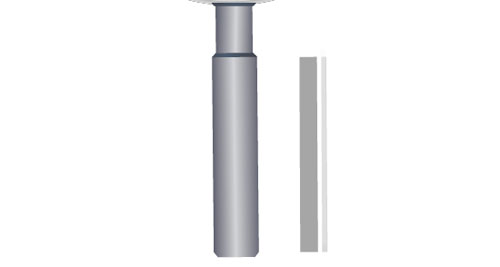46. Fill the shapes with white color and set opacity to 20% then place them over the handle.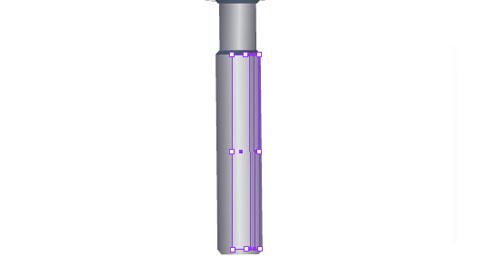47. After placing the shape, the basic structure of the glass is completed. Make one more circle inside the magnifying glass, as seen in the image below.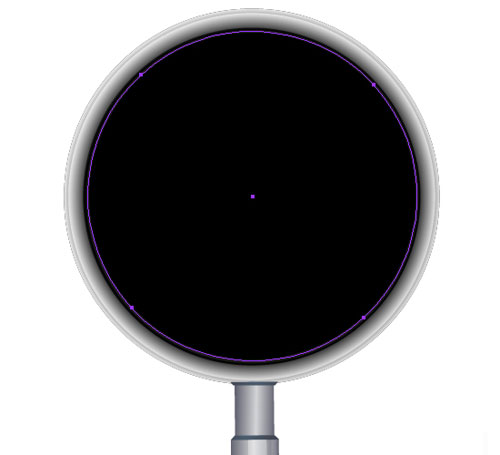48. Now, we’ll place the compass under the magnifying glass shape, as shown in the image below.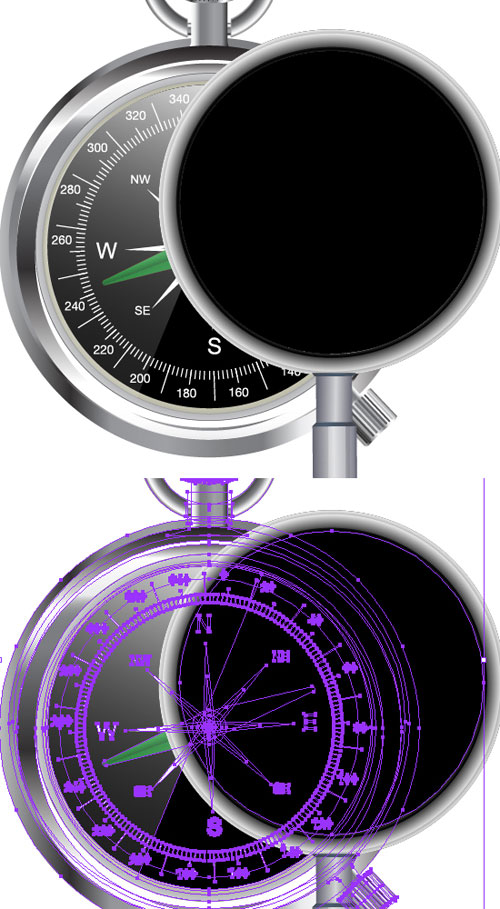49. Select the magnifying glass circle and the compass then go to Object > Clipping Mask > Make.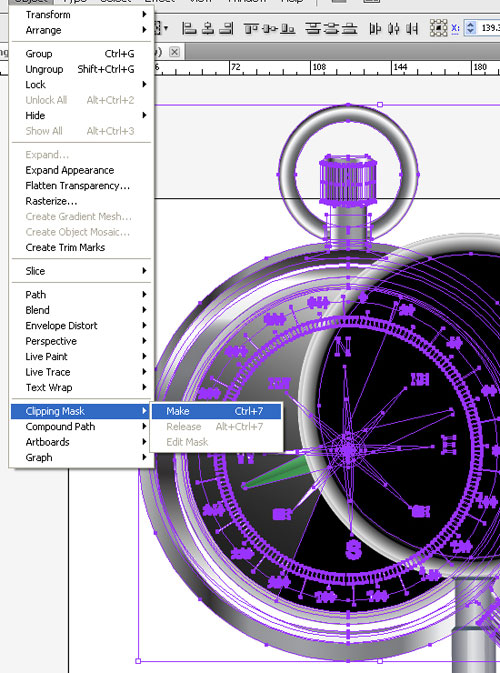50. By doing the clip mask, the compass will be masked with the circle.51. We will now place the magnifying glass over the compass, but remember that placement should be exact.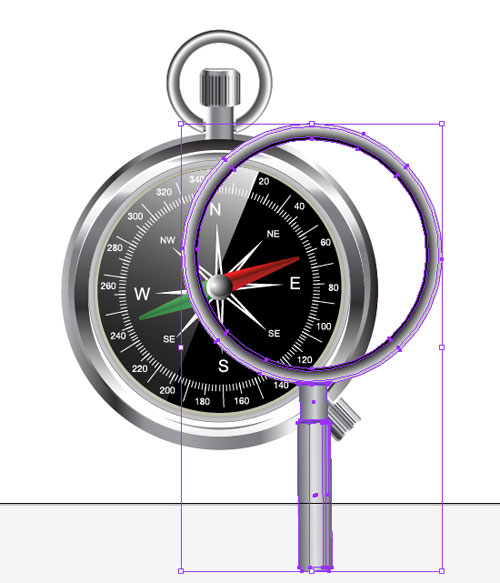52. Rotate the glass as per your liking. Remember that you have to rotate the glass, but not the clipping mask of the compass. See the image below.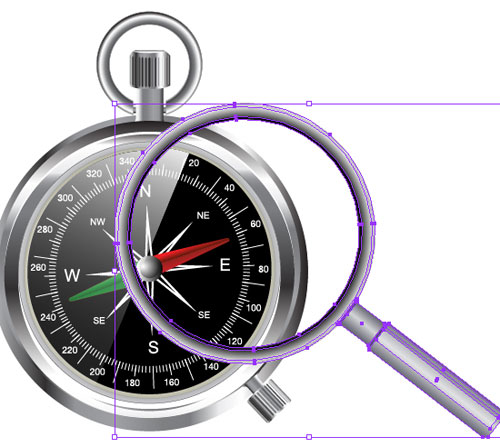53. We’ll go inside the clipping mask of the compass and increase the size of the compass by 30%. To increase the size, go to clipping mask and select the compass; then go to toolbox and double click the tool marked red.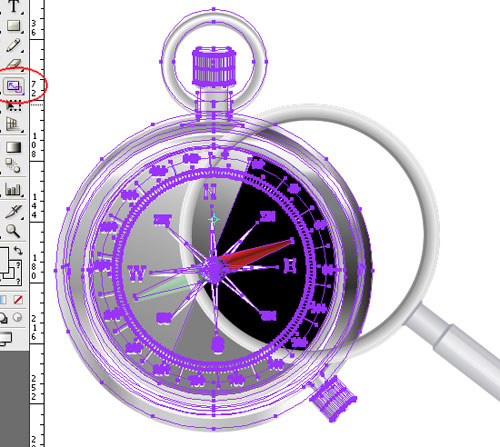54. By double clicking, you will see a window in front of you. Input the values given below then press OK.55. After pressing OK, the size of the compass will increase.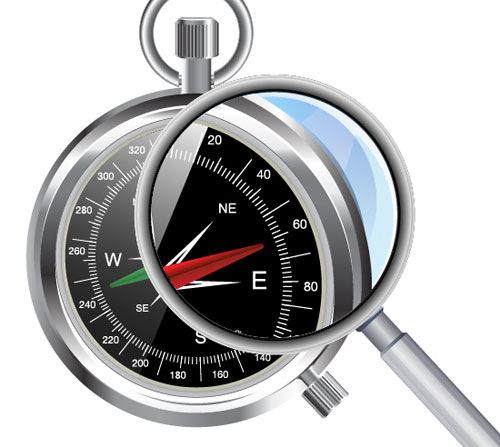56. Now, move the magnifying glass, with it’s clipping mask, slightly to the upper right side of the compass.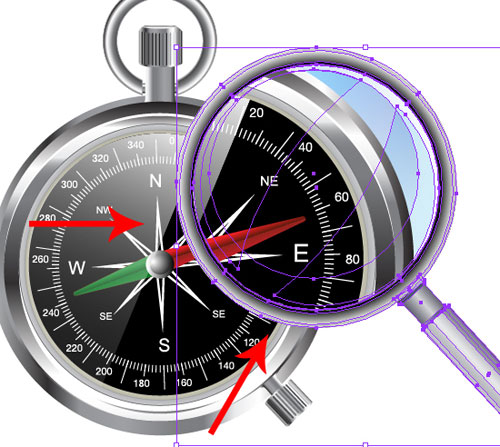57. The final image is now complete and looks like this.

### Final Image